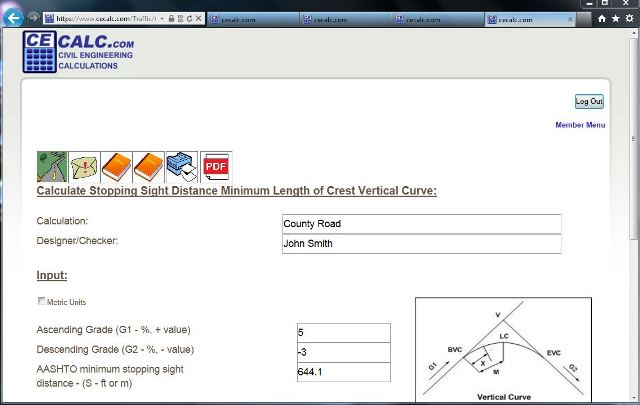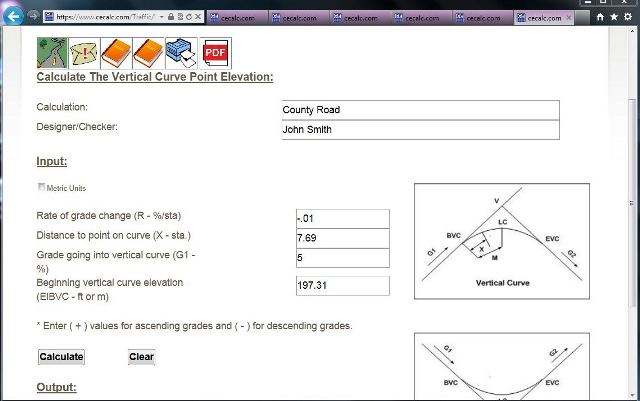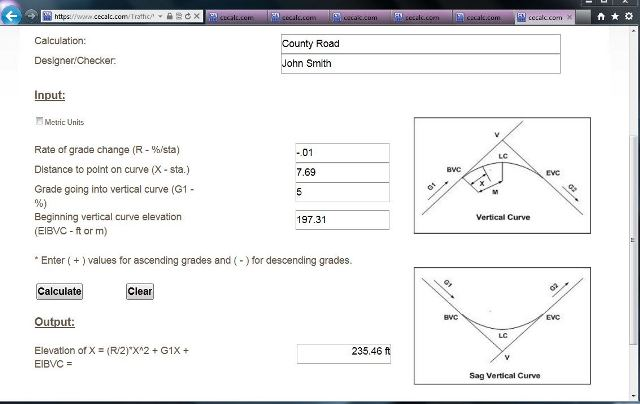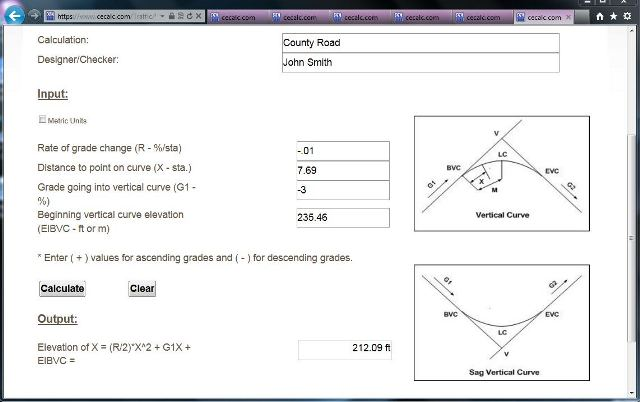## Transportation Design Problem 2 - Determine elevation of a point on a crest vertical curve:

Given a crest vertical curve connecting an ascending grade of 5% to a descending grade of 3%. The PVC at station 23+00 has an elevation of 197.31. If the vertical curve is designed for a stopping distance of 644.1 feet at 65 mph, what are the elevations of the PVI and PVT?
##### 1.
From the transportation submenu open the length crest vertical curve stopping sight distance calculation, CrstVrtCrvStop.aspx. Input the "Title" of the calculation, the "Designer/Checker" information and the variables.  Click on the "Calculate" button. Read the results. The length of the crest vertical curve based on stopping sight distance is 1537.96 feet.##### 2.
From the transportation submenu open the rate of grade change calculation, GrdChngRt.aspx. Input the "Title" of the calculation, the "Designer/Checker" information and the variables.  Click on the "Calculate" button. Read the results. The rate of grade change is -0.01 %/ft.##### 3.
From the transportation submenu open the vertical curve point elevation calculation, VertCrvPntEl.aspx. Input the "Title" of the calculation, the "Designer/Checker" information and the variables.  The distance to PVI is LC/2 = 1537.96/2 = 768.98 feet. Enter 7.69 for distance in stations. Click on the "Calculate" button. Read the results. The elevation of the PVI is 235.46 feet.##### 4.
Still working in the vertical curve point elevation calculation, VertCrvPntEl.aspx. Input any changes to the "Title" of the calculation, the "Designer/Checker" information.  Change the input variables. For grade entering the vertical curve enter the descending grade of -3%. Change the beginning vertical curve elevation to that of the PVI elevation of 235.46. Click on the "Calculate" button. Read the results. The elevation of the PVT is 212.09 feet.##### 5. Summary
The elevation of the PVI is 235.46 feet. The elevation of the PVT is 212.09 feet.

 Start Using The Calculations >>> Become A Member Now!